MORE IN Engineering Mechanics (EM)
SPPU First Year Engineering (Semester 2)
Engineering Mechanics (EM)
June 2015
Total marks: --
Total time: --
INSTRUCTIONS
(1) Assume appropriate data and state your reasons
(2) Marks are given to the right of every question
(3) Draw neat diagrams wherever necessary

Answer any one question from Q1 and Q2
1 (a) Combine the two forces 800 N and 600 N, which act on the fixed dam structure at B, into a single equivalent force R if AC = 3 m, BC = 6 m and angle BCD = 60°. Refer Fig. 1(a):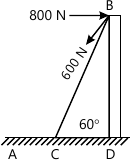4 M
1 (b) A 50 kg crate shown in Fig. 1(b) rests on a horizontal plane for which the coefficient of kinetic friction is μk = 0.3. If the crate does not tip over when it is subjected to a 400 N towing force as shown, determine the velocity of the crate in 5 s starting from rest.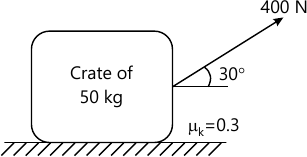4 M
1 (c) A projectile is fired from the edge of a 150 m cliff with an initial velocity of 180 m/s at an angle of 30° with the horizontal. Neglecting air resistance, find the horizontal distance from the gun to the point, where the projectile strikes the ground and the greatest elevation above the ground reached by the projectile. Refer Fig. 1 (c)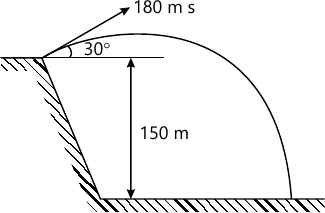4 M
1 (d) A 2 kg stone is dropped from a height h and strikes the ground with a velocity of 24m/s. Find the kinetic energy of the stone as it strikes the ground and the height h from which it was dropped.
4 M

2 (a) Determine the x coordinates of the centroid with respect to the origin O of the shaded area as shown in Fig. 2(a):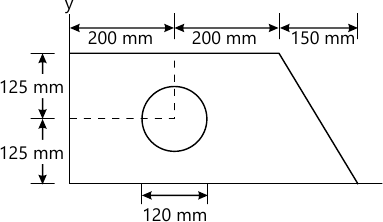4 M
2 (b) A car comes to complete stop from an initial speed of 50 kmph in a distance of 100 m. With the same constant acceleration, what would be the stopping distance s from an initial speed of 70 kmph.
4 M
2 (c) If the crest of the hill has a radius of curvature ρ= 60 m, determine the maximum constant speed at which the car of weight 17.5 kN can travel over it without leaving the surface of the road.
4 M
2 (d) A 1.5 kg collar is attached to a spring and slides without friction along a circular rod in a horizontal plane. The spring has an undeformed length of 150 mm and a constant k = 400 N/m. Knowing that the collar is in equilibrium at A and is given a slight push to get moving, determine the velocity of the collar as it passes point B. Refer 2(d):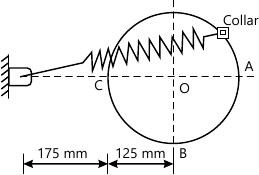4 M

Answer any one question from Q3 and Q4
3 (a) Determine the resultant of the parallel force system which act on the plate as shown in Fig. 3(a):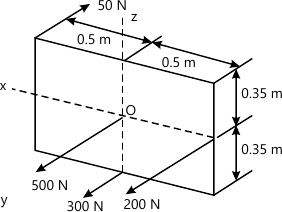6 M
3 (b) The boom is intended to support two vertical loads F1 and F2 as shown in Fig. 3(b). If the cable CB can sustain a maximum load of 1500 N before it fails. Determine the critical loads if F1 = 2F2. Also determine the reaction at pin support A.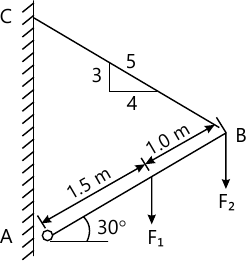6 M
3 (c) Determine the support reaction of the beam loaded and supported as shown in Fig. 3(c):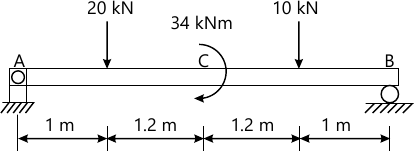5 M

4 (a) The square steel plate has a mass of 1500 kg with mass center at its center G. Calculate the tension in each of the three cables with which the plate is lifted while remaining horizontal. Refer Fig. 4 (a).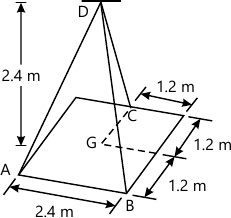6 M
4 (b) The 20 kg homogeneous smooth sphere rests on the two inclines as shown in Fig. (b). Determine the contact forces at A and B.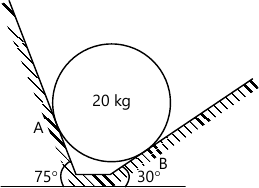6 M
4 (c) A beam supported a load varying uniformly from a intensity of w1 kN/m at left end to w2 kN/m at the right end as shown in Fig. 4 (c). If the reactions RL=6kN and RR=12 kN, determine the intensity of loading w1 and w2: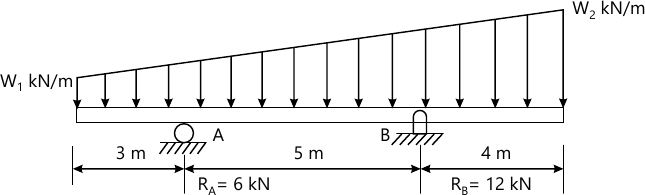5 M

Answer any one question from Q5 and Q6
5 (a) Determine the force in members FE, FC and BC of the truss using method of sections and state if the members are in tension or compression. Given P1 = 2.22 kN and P2 = 6.66 kN. Refer Fig. 5 (a).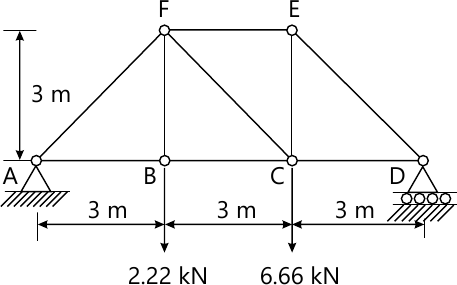6 M
5 (b) Cable ABCD supports the 4 kN and 6 kN loads at points B and C as shown in Fig. 5 (b). Determine the maximum tension in cable and the sag of point B.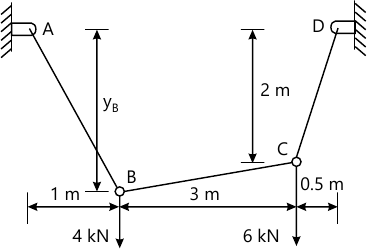5 M
5 (c) Determine the distance 's' to which the 90 kg man can climb without causing the 4m ladder to slip at its lower end. The top of the 15 kg ladder has a small roller and at the ground the coefficient of static friction is μs = 0.25. The mass centre of the man is directly above his feet.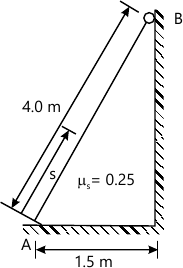6 M

6 (a) Determine the horizontal force P needed to just start moving the 300N create up the plane as shown in Fig. 6(a). Take μs=0.1.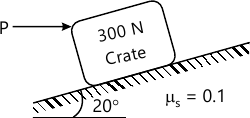6 M
6 (b) Determine the minimum coefficient of static friction between the rope and the fixed shaft as shown in Fig. 6 (b) will prevent the unbalanced cylinder from moving.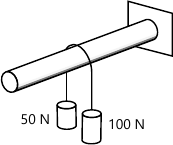5 M
6 (c) Determine the reactions at the internal hinge C for the frame loaded and supported as shown in Fig. 6(c).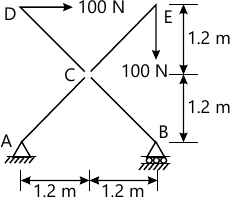6 M

More question papers from Engineering Mechanics (EM)